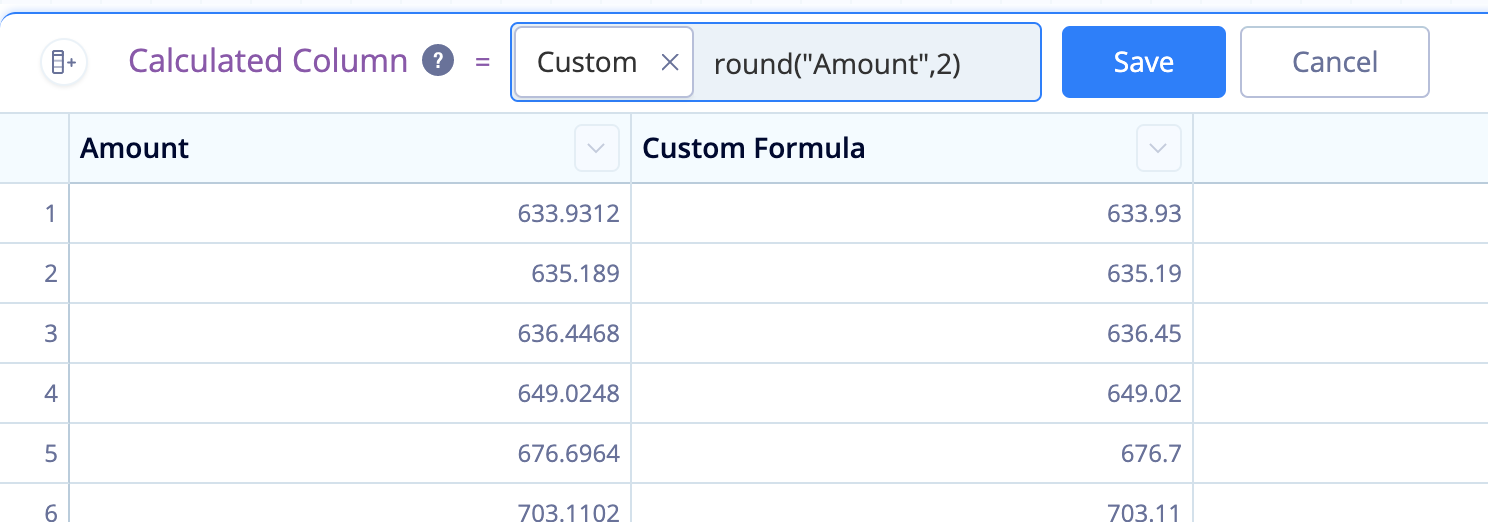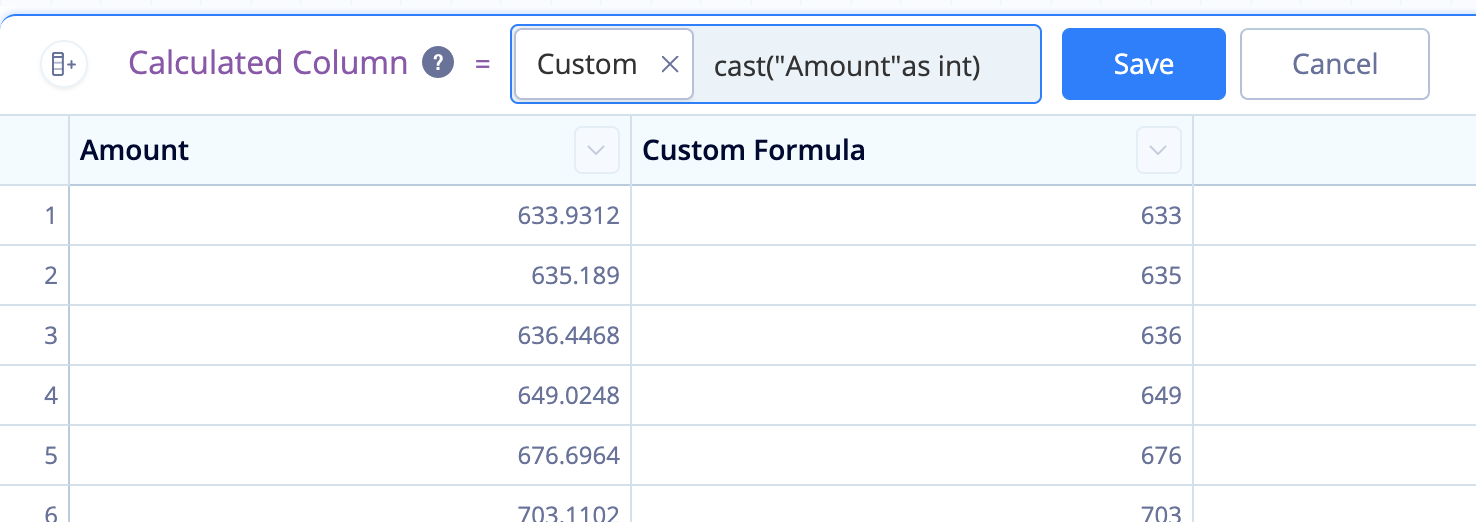# Round numbers in Visual SQL

The Formula Column and Apply Formula Actions allow you to perform functions on your data, including our custom functions, standard SQLite functions, and the following math functions: `degrees()`, `radians()`, `cos()`, `sin()`, `tan()`, `cosh()`, `sinh()`, `tanh()`, `exp()`, `log()`, `log10()`, `sqrt()`, `ceil()`, `floor()`

Check out the full list of SQLite functions to see what other transformations are possible.

## Round function

If you’re performing a calculation on your data, you’ll often need to round the resulting numbers, which you can do using the `round()` function.

With your chart open in Visual SQL, click Formula Column above the Result Table, select the Custom formula type, and use the following formula:

``````round("Column Name", 2)
``````

where `Column Name` is the name of the column you’d like to round, and `2` is the number of decimal points you’d like to round up to in your column.

Click Save to apply the changes and keep the pipeline step open to preview your data and see the transformation. Click Apply & Close to apply the changes and close the Pipeline step.If you’re performing a calculation on integers, then only integers will be returned. If you want your results to have decimal precision, you’ll need to convert your values to decimals before performing any calculations.

You can do this by using the Apply Formula Action. Use a Custom formula and multiply your formula numerator by `1.0`. For example:

``````(1.0 * "Column 1")/"Column 2"
``````

Multiplying “Column 1” by `1.0` will force the value to be converted to the data type “real” and show decimal precision.

You can also do this directly within the round() function as shown in the example below:

``````round(1.0 * "Column 1" / "Column 2", 2)
``````

## Casting the column as an integer

If you want to remove all decimals from your values, you can also use the `cast()` function to cast your column as an integer. This will convert all the values into whole numbers.

Adding either a Formula Column or Apply Formula Action, use a Custom formula and enter the following formula:

``````cast("Column Name" as int)
``````

where `Column Name` is the name of your original column.

Note: This doesn’t round to the nearest whole number; it simply removes the decimal point and its trailing numbers.